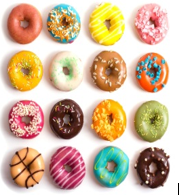RB 3rd Multiplication and Division Word Problems
starstarstarstarstarstarstarstarstarstar
1 (5 ratings)
by SAMANTHA WILLIAMS
| 11 Questions
1
1 pt
Write the related facts for 6, 7, and 32.2
1 pt
Write a multiplication and division equation for the array above.
3
1 pt
_____ x 6 = 18
What division equation can you use to solve the equation above?
4
1 pt
4 x ____= 24
24/ 4 =____
Which number fits in both blanks?
5
1 pt
5 x ____ = 20
20/5 = _____
6
1 pt
32/____ = 8
What multiplication equation can you use to solve the equation above?
7
1 pt
Jan and Jon pick 18 apples. They share them equally. Whick facts can be used to find the number of apples each person gets? Select the 2 answers that apply.
A. 6 x 3 = 18
B. 2 x 9 = 18
C. 18/2 =9
D. 18/3 =6
E. 18/9 =2

8
1 pt
Which fact can you use to solve 5 = 20/?
A. 5 x 5 = 25
B. 4 x 5 = 20
C. 5 + 15 = 20
D. 6 x 4 =24

9
1 pt
42/6 = 2 + _____
A. 4
B. 5
C. 7
D. 9

10
1 pt
Which three numbers can be in a related fact family?
A. 3, 4, 15
B. 2, 7, 18
C. 9, 3, 27
D

11
1 pt
Which 2 problems can be solved with the multiplication equation 7 x 8 = 56? Select all the correct answers.
A. Sofia has 56 balloons. She gives 8 balloons to her friend. How many balloons does Sofia have now?
B. Devon has 7 baskets. He puts 8 toys in each basket. What is the total number of toys Devon puts in the baskets?
C. There ate 56 tables in the lunchroom. Each table has 8 chairs. How many chairs are their in all?
D. Lisa walks 8 blocks to her friend's house. Then she walks 7 more blocks to school. How many blocks does Lisa walk in all?
E. Juan puts his baseball cards into packs of 8 cards each. He has 7 packs of cards. How many baseball cards does Juan have?

Add to my formatives list